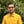Trusted answers to developer questions
Trusted Answers to Developer Questions

Related Tags

d
acosh
communitycreator

# What is the acosh() function in D?Harris Amjad

Grokking Modern System Design Interview for Engineers & Managers

Ace your System Design Interview and take your career to the next level. Learn to handle the design of applications like Netflix, Quora, Facebook, Uber, and many more in a 45-min interview. Learn the RESHADED framework for architecting web-scale applications by determining requirements, constraints, and assumptions before diving into a step-by-step design process.

### Overview

The acosh() function returns the inverse hyperbolic cosine of a number.

We can see the mathematical representation of the acosh() function below.

$acosh(x) =ln(x+√(x^{2}-1))$

Note: std.math is required for this function.

### Syntax

acosh(number)  //number can be real, float, or double.


### Parameters

This function requires a number as a parameter. The value of the parameter should be greater than or equal to 1.

### Return value

acosh() returns the inverse hyperbolic cosine of a number that is sent as a parameter.

### Code

The following code shows how to use the acosh() function in D.

import core.stdc.stdio;import std.stdio;//header required for functionimport std.math;int main() {    //positive number     writeln("The value of acosh(1.5) :", acosh(1.5));    // 1    writeln("The value of acosh(1.0) :", acosh(1.0));    //less than 1    writeln("The value of acosh(0.0): ",acosh(0.0) );    writeln("The value of acosh(-1.5): ",acosh(-1.5) );    return 0;}

### Explanation

• Line 4: We add the std.math header required for acosh() function.
• Line 8: We calculate the inverse hyperbolic cosine of the positive number greater than 1 using acosh().
• Line 11: We calculate inverse hyperbolic cosine of 1 using acosh().
• Lines 14–15: We calculate the inverse hyperbolic cosine of the number less than 1 using acosh().

RELATED TAGS

d
acosh
communitycreator

CONTRIBUTORHarris Amjad

Grokking Modern System Design Interview for Engineers & Managers

Ace your System Design Interview and take your career to the next level. Learn to handle the design of applications like Netflix, Quora, Facebook, Uber, and many more in a 45-min interview. Learn the RESHADED framework for architecting web-scale applications by determining requirements, constraints, and assumptions before diving into a step-by-step design process.

Keep Exploring

Learn in-demand tech skills in half the time